# Generation module#

Functions that generates the underlying connectivity of graphs, as well as the connection properties (weight/strength and delay).

## Content#

### Generation functions#

 nngt.generation.all_to_all([nodes, ...]) Generate a graph where all nodes are connected. nngt.generation.circular(coord_nb[, ...]) Generate a circular graph. nngt.generation.distance_rule(scale[, rule, ...]) Create a graph using a 2D distance rule to create the connection between neurons. nngt.generation.erdos_renyi([density, ...]) Generate a random graph as defined by Erdos and Renyi but with a reciprocity that can be chosen. nngt.generation.fixed_degree(degree[, ...]) Generate a random graph with constant in- or out-degree. nngt.generation.from_degree_list(degrees[, ...]) Generate a random graph from a given list of degrees. nngt.generation.gaussian_degree(avg, std[, ...]) Generate a random graph with constant in- or out-degree. nngt.generation.newman_watts(coord_nb[, ...]) Generate a (potentially small-world) graph using the Newman-Watts algorithm. nngt.generation.price_scale_free(m[, c, ...]) Generate a Price graph model (Barabasi-Albert if undirected). nngt.generation.random_scale_free(in_exp, ...) Generate a free-scale graph of given reciprocity and otherwise devoid of correlations. nngt.generation.sparse_clustered(c[, nodes, ...]) Generate a sparse random graph with given average clustering coefficient and degree. nngt.generation.watts_strogatz(coord_nb[, ...]) Generate a (potentially small-world) graph using the Watts-Strogatz algorithm.

### Connectors#

 nngt.generation.connect_nodes(network, ...) Function to connect nodes with a given graph model. nngt.generation.connect_groups(network, ...) Function to connect groups with a given graph model. nngt.generation.connect_neural_types(...[, ...]) Function to connect excitatory and inhibitory population with a given graph model.

### Rewiring functions#

 nngt.generation.random_rewire(g[, ...]) Generate a new rewired graph from g. nngt.generation.lattice_rewire(g[, ...]) Build a (generally irregular) lattice by rewiring the edges of a graph.

## Details#

nngt.generation.all_to_all(nodes=0, weighted=True, directed=True, multigraph=False, name='AllToAll', shape=None, positions=None, population=None, **kwargs)[source]#

Generate a graph where all nodes are connected.

New in version 1.0.

Parameters:
• nodes (int, optional (default: None)) – The number of nodes in the graph.

• reciprocity (double, optional (default: -1 to let it free)) – Fraction of edges that are bidirectional (only for directed graphs – undirected graphs have a reciprocity of 1 by definition)

• weighted (bool, optional (default: True)) – Whether the graph edges have weights.

• directed (bool, optional (default: True)) – Whether the graph is directed or not.

• multigraph (bool, optional (default: False)) – Whether the graph can contain multiple edges between two nodes.

• name (string, optional (default: “ER”)) – Name of the created graph.

• shape (Shape, optional (default: None)) – Shape of the neurons’ environment.

• positions (numpy.ndarray, optional (default: None)) – A 2D or 3D array containing the positions of the neurons in space.

• population (NeuralPop, optional (default: None)) – Population of neurons defining their biological properties (to create a Network).

Note

nodes is required unless population is provided.

Returns:

graph_all (Graph, or subclass) – A new generated graph.

nngt.generation.circular(coord_nb, reciprocity=1.0, reciprocity_choice='random', nodes=0, weighted=True, directed=True, multigraph=False, name='Circular', shape=None, positions=None, population=None, from_graph=None, **kwargs)[source]#

Generate a circular graph.

The nodes are placed on a circle and connected to their coord_nb closest neighbours. If the graph is directed, the number of connections depends on the value of reciprocity: if reciprocity == 0., then only half of all possible connections will be created, so that no bidirectional edges exist; on the other hand, for reciprocity == 1., all possible edges are created; for intermediate values of reciprocity, the number of edges increases linearly as 0.5*(1 + reciprocity / (2 - reciprocity))*nodes*coord_nb.

Parameters:
• coord_nb (int) – The number of neighbours for each node on the initial topological lattice (must be even).

• reciprocity (double, optional (default: 1.)) – Proportion of reciprocal edges in the graph.

• reciprocity_choice (str, optional (default: “random”)) – How reciprocal edges should be chosen, which can be either “random” or “closest”. If the latter option is used, then connections between first neighbours are rendered reciprocal first, then between second neighbours, etc.

• nodes (int, optional (default: None)) – The number of nodes in the graph.

• density (double, optional (default: 0.1)) – Structural density given by edges / (nodes*nodes).

• edges (int (optional)) – The number of edges between the nodes

• avg_deg (double, optional) – Average degree of the neurons given by edges / nodes.

• weighted (bool, optional (default: True)) – Whether the graph edges have weights.

• directed (bool, optional (default: True)) – Whether the graph is directed or not.

• multigraph (bool, optional (default: False)) – Whether the graph can contain multiple edges between two nodes.

• name (string, optional (default: “ER”)) – Name of the created graph.

• shape (Shape, optional (default: None)) – Shape of the neurons’ environment

• positions (numpy.ndarray, optional (default: None)) – A 2D or 3D array containing the positions of the neurons in space.

• population (NeuralPop, optional (default: None)) – Population of neurons defining their biological properties (to create a Network).

• from_graph (Graph or subclass, optional (default: None)) – Initial graph whose nodes are to be connected.

Returns:

graph_circ (Graph or subclass)

nngt.generation.connect_groups(network, source_groups, target_groups, graph_model, density=None, edges=None, avg_deg=None, unit='um', weighted=True, directed=True, multigraph=False, check_existing=True, ignore_invalid=False, **kwargs)[source]#

Function to connect groups with a given graph model.

Changed in version 2.0: Added check_existing and ignore_invalid arguments.

Parameters:
• network (Network or SpatialNetwork) – The network to connect.

• source_groups (str, NeuralGroup, or iterable) – Names of the source groups (which contain the pre-synaptic neurons) or directly the group objects themselves.

• target_groups (str, NeuralGroup, or iterable) – Names of the target groups (which contain the post-synaptic neurons) or directly the group objects themselves.

• graph_model (string) – The name of the connectivity model (among “erdos_renyi”, “random_scale_free”, “price_scale_free”, and “newman_watts”).

• check_existing (bool, optional (default: True)) – Check whether some of the edges that will be added already exist in the graph.

• ignore_invalid (bool, optional (default: False)) – Ignore invalid edges: they are not added to the graph and are silently dropped. Unless this is set to true, an error is raised if an existing edge is re-generated.

• kwargs (keyword arguments) – Specific model parameters. or edge attributes specifiers such as weights or delays.

Note

For graph generation methods which set the properties of a specific degree (e.g. gaussian_degree()), the groups which have their property sets are the source_groups.

nngt.generation.connect_neural_groups(*args, **kwargs)[source]#

Deprecatd alias of connect_groups().

nngt.generation.connect_neural_types(network, source_type, target_type, graph_model, density=None, edges=None, avg_deg=None, unit='um', weighted=True, directed=True, multigraph=False, check_existing=True, ignore_invalid=False, **kwargs)[source]#

Function to connect excitatory and inhibitory population with a given graph model.

Changed in version 2.0: Added check_existing and ignore_invalid arguments.

Parameters:
• network (Network or SpatialNetwork) – The network to connect.

• source_type (int or list) – The type of source neurons (1 for excitatory, -1 for inhibitory neurons).

• target_type (int or list) – The type of target neurons.

• graph_model (string) – The name of the connectivity model (among “erdos_renyi”, “random_scale_free”, “price_scale_free”, and “newman_watts”).

• check_existing (bool, optional (default: True)) – Check whether some of the edges that will be added already exist in the graph.

• ignore_invalid (bool, optional (default: False)) – Ignore invalid edges: they are not added to the graph and are silently dropped. Unless this is set to true, an error is raised if an existing edge is re-generated.

• kwargs (keyword arguments) – Specific model parameters. or edge attributes specifiers such as weights or delays.

Note

For graph generation methods which set the properties of a specific degree (e.g. gaussian_degree()), the nodes which have their property sets are the source_type.

nngt.generation.connect_nodes(network, sources, targets, graph_model, density=None, edges=None, avg_deg=None, unit='um', weighted=True, directed=True, multigraph=False, check_existing=True, ignore_invalid=False, **kwargs)[source]#

Function to connect nodes with a given graph model.

Changed in version 2.0: Added check_existing and ignore_invalid arguments.

Parameters:
• network (Network or SpatialNetwork) – The network to connect.

• sources (list) – Ids of the source nodes.

• targets (list) – Ids of the target nodes.

• graph_model (string) – The name of the connectivity model (among “erdos_renyi”, “random_scale_free”, “price_scale_free”, and “newman_watts”).

• check_existing (bool, optional (default: True)) – Check whether some of the edges that will be added already exist in the graph.

• ignore_invalid (bool, optional (default: False)) – Ignore invalid edges: they are not added to the graph and are silently dropped. Unless this is set to true, an error is raised if an existing edge is re-generated.

• **kwargs (keyword arguments) – Specific model parameters. or edge attributes specifiers such as weights or delays.

Note

For graph generation methods which set the properties of a specific degree (e.g. gaussian_degree()), the nodes which have their property sets are the sources.

nngt.generation.distance_rule(scale, rule='exp', shape=None, neuron_density=1000.0, max_proba=-1.0, nodes=0, density=None, edges=None, avg_deg=None, unit='um', weighted=True, directed=True, multigraph=False, name='DR', positions=None, population=None, from_graph=None, **kwargs)[source]#

Create a graph using a 2D distance rule to create the connection between neurons. Available rules are linear and exponential.

Parameters:
• scale (float) – Characteristic scale for the distance rule. E.g for linear distance- rule,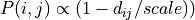, whereas for the exponential distance-rule,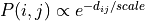.

• rule (string, optional (default: ‘exp’)) – Rule that will be apply to draw the connections between neurons. Choose among “exp” (exponential), “gaussian” (Gaussian), or “lin” (linear).

• shape (Shape, optional (default: None)) – Shape of the neurons’ environment. If not specified, a square will be created with the appropriate dimensions for the number of neurons and the neuron spatial density.

• neuron_density (float, optional (default: 1000.)) – Density of neurons in space (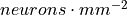).

• nodes (int, optional (default: None)) – The number of nodes in the graph.

• p (float, optional) – Normalization factor for the distance rule; it is equal to the probability of connection when testing a node at zero distance.

• density (double, optional) – Structural density given by edges / (nodes * nodes).

• edges (int, optional) – The number of edges between the nodes

• avg_deg (double, optional) – Average degree of the neurons given by edges / nodes.

• unit (string (default: ‘um’)) – Unit for the length scale among ‘um’ (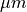), ‘mm’, ‘cm’, ‘dm’, ‘m’.

• weighted (bool, optional (default: True)) – Whether the graph edges have weights.

• directed (bool, optional (default: True)) – Whether the graph is directed or not.

• multigraph (bool, optional (default: False)) – Whether the graph can contain multiple edges between two nodes.

• name (string, optional (default: “DR”)) – Name of the created graph.

• positions (numpy.ndarray, optional (default: None)) – A 2D (N, 2) or 3D (N, 3) shaped array containing the positions of the neurons in space.

• population (NeuralPop, optional (default: None)) – Population of neurons defining their biological properties (to create a Network).

• from_graph (Graph or subclass, optional (default: None)) – Initial graph whose nodes are to be connected.

nngt.generation.erdos_renyi(density=None, nodes=0, edges=None, avg_deg=None, reciprocity=-1.0, weighted=True, directed=True, multigraph=False, name='ER', shape=None, positions=None, population=None, from_graph=None, **kwargs)[source]#

Generate a random graph as defined by Erdos and Renyi but with a reciprocity that can be chosen.

Parameters:
• density (double, optional (default: -1.)) – Structural density given by edges / nodes. It is also the probability for each possible edge in the graph to exist.

• nodes (int, optional (default: None)) – The number of nodes in the graph.

• edges (int (optional)) – The number of edges between the nodes

• avg_deg (double, optional) – Average degree of the neurons given by edges / nodes.

• reciprocity (double, optional (default: -1 to let it free)) – Fraction of edges that are bidirectional (only for directed graphs – undirected graphs have a reciprocity of 1 by definition)

• weighted (bool, optional (default: True)) – Whether the graph edges have weights.

• directed (bool, optional (default: True)) – Whether the graph is directed or not.

• multigraph (bool, optional (default: False)) – Whether the graph can contain multiple edges between two nodes.

• name (string, optional (default: “ER”)) – Name of the created graph.

• shape (Shape, optional (default: None)) – Shape of the neurons’ environment.

• positions (numpy.ndarray, optional (default: None)) – A 2D or 3D array containing the positions of the neurons in space.

• population (NeuralPop, optional (default: None)) – Population of neurons defining their biological properties (to create a Network).

• from_graph (Graph or subclass, optional (default: None)) – Initial graph whose nodes are to be connected.

Returns:

graph_er (Graph, or subclass) – A new generated graph or the modified from_graph.

Note

nodes is required unless from_graph or population is provided. If an from_graph is provided, all preexistant edges in the object will be deleted before the new connectivity is implemented.

nngt.generation.fixed_degree(degree, degree_type='in', nodes=0, reciprocity=-1.0, weighted=True, directed=True, multigraph=False, name='FD', shape=None, positions=None, population=None, from_graph=None, **kwargs)[source]#

Generate a random graph with constant in- or out-degree.

Parameters:
• degree (int) – The value of the constant degree.

• degree_type (str, optional (default: ‘in’)) – The type of the fixed degree, among 'in', 'out' or 'total'.

• nodes (int, optional (default: None)) – The number of nodes in the graph.

• reciprocity (double, optional (default: -1 to let it free)) – @todo: not implemented yet. Fraction of edges that are bidirectional (only for directed graphs – undirected graphs have a reciprocity of 1 by definition)

• weighted (bool, optional (default: True)) – Whether the graph edges have weights.

• directed (bool, optional (default: True)) – @todo: only for directed graphs for now. Whether the graph is directed or not.

• multigraph (bool, optional (default: False)) – Whether the graph can contain multiple edges between two nodes.

• name (string, optional (default: “ER”)) – Name of the created graph.

• shape (Shape, optional (default: None)) – Shape of the neurons’ environment.

• positions (numpy.ndarray, optional (default: None)) – A 2D or 3D array containing the positions of the neurons in space.

• population (NeuralPop, optional (default: None)) – Population of neurons defining their biological properties (to create a Network).

• from_graph (Graph or subclass, optional (default: None)) – Initial graph whose nodes are to be connected.

Note

nodes is required unless from_graph or population is provided. If an from_graph is provided, all preexistant edges in the object will be deleted before the new connectivity is implemented.

Returns:

graph_fd (Graph, or subclass) – A new generated graph or the modified from_graph.

nngt.generation.from_degree_list(degrees, degree_type='in', weighted=True, directed=True, multigraph=False, name='DL', shape=None, positions=None, population=None, from_graph=None, **kwargs)[source]#

Generate a random graph from a given list of degrees.

Parameters:
• degrees (list) – The list of degrees for each node in the graph.

• degree_type (str, optional (default: ‘in’)) – The type of the fixed degree, among 'in', 'out' or 'total'.

• nodes (int, optional (default: None)) – The number of nodes in the graph.

• weighted (bool, optional (default: True)) – Whether the graph edges have weights.

• directed (bool, optional (default: True)) – Whether the graph is directed or not.

• multigraph (bool, optional (default: False)) – Whether the graph can contain multiple edges between two nodes.

• name (string, optional (default: “ER”)) – Name of the created graph.

• shape (Shape, optional (default: None)) – Shape of the neurons’ environment.

• positions (numpy.ndarray, optional (default: None)) – A 2D or 3D array containing the positions of the neurons in space.

• population (NeuralPop, optional (default: None)) – Population of neurons defining their biological properties (to create a Network).

• from_graph (Graph or subclass, optional (default: None)) – Initial graph whose nodes are to be connected.

Returns:

graph_dl (Graph, or subclass) – A new generated graph or the modified from_graph.

nngt.generation.gaussian_degree(avg, std, degree_type='in', nodes=0, reciprocity=-1.0, weighted=True, directed=True, multigraph=False, name='GD', shape=None, positions=None, population=None, from_graph=None, **kwargs)[source]#

Generate a random graph with constant in- or out-degree.

Parameters:
• avg (float) – The value of the average degree.

• std (float) – The standard deviation of the Gaussian distribution.

• degree_type (str, optional (default: ‘in’)) – The type of the fixed degree, among ‘in’, ‘out’ or ‘total’ (or the full version: ‘in-degree’…) @todo: Implement ‘total’ degree

• nodes (int, optional (default: None)) – The number of nodes in the graph.

• reciprocity (double, optional (default: -1 to let it free)) – @todo: not implemented yet. Fraction of edges that are bidirectional (only for directed graphs – undirected graphs have a reciprocity of 1 by definition)

• weighted (bool, optional (default: True)) – Whether the graph edges have weights.

• directed (bool, optional (default: True)) – @todo: only for directed graphs for now. Whether the graph is directed or not.

• multigraph (bool, optional (default: False)) – Whether the graph can contain multiple edges between two nodes.

• name (string, optional (default: “ER”)) – Name of the created graph.

• shape (Shape, optional (default: None)) – Shape of the neurons’ environment.

• positions (numpy.ndarray, optional (default: None)) – A 2D or 3D array containing the positions of the neurons in space.

• population (NeuralPop, optional (default: None)) – Population of neurons defining their biological properties (to create a Network).

• from_graph (Graph or subclass, optional (default: None)) – Initial graph whose nodes are to be connected.

Returns:

graph_gd (Graph, or subclass) – A new generated graph or the modified from_graph.

Note

nodes is required unless from_graph or population is provided. If an from_graph is provided, all preexistant edges in the object will be deleted before the new connectivity is implemented.

nngt.generation.lattice_rewire(g, target_reciprocity=1.0, node_attr_constraints=None, edge_attr_constraints=None, weight=None, weight_constraint='distance', distance_sort='inverse')[source]#

Build a (generally irregular) lattice by rewiring the edges of a graph.

New in version 2.0.

The lattice is based on a circular graph, meaning that the nodes are placed on a circle and connected based on the topological distance between them, the distance being defined through the positive modulo: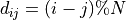with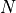the number of nodes in the graph.

Parameters:
• g (Graph) – Graph based on which the lattice will be generated.

• target_reciprocity (float, optional (default: 1.)) – Value of reciprocity that should be aimed at. Depending on the number of edges, it may not be possible to reach this value exactly.

• node_attr_constraints (str, optional (default: randomize all attributes)) – Whether attribute randomization is constrained: either “preserve”, where all nodes keep their attributes, or “together”, where attributes are randomized by groups (all attributes of a given node are sent to the same new node). By default, attributes are completely and separately randomized.

• edge_attr_constraints (str, optional (default: randomize all but weight)) – Whether attribute randomization is constrained. If “distance” is used, then all number attributes (float or int) are sorted and are first associated to the shortest or longest edges depending on the value of distance_sort. Note that, for directed graphs, if a reciprocal edge exists, it is immediately assigned the next highest (respectively lowest) attribute after that of its directed couterpart. If “together” is used, edges attributes are randomized by groups (all attributes of a given edge are sent to the same new edge) either randomly if weight is None, or following the constrained weight attribute. By default, attributes are completely and separately randomized (except for weight if it has been provided).

• weight (str, optional (default: None)) – Whether a specific edge attribute should play the role of weight and have special constraints.

• weight_constraint (str, optional (default: “distance”)) – Same as edge_attr_constraints but only applies to weight and can only be “distance” or None since “together” was related to weight.

• distance_sort (str, optional (default: “inverse”)) – How attributes are sorted with edge distance: either “inverse”, with the shortest edges being assigned the largest weights, or with a “linear” sort, where shortest edges are assigned the lowest weights.

nngt.generation.newman_watts(coord_nb, proba_shortcut=None, reciprocity_circular=1.0, reciprocity_choice_circular='random', nodes=0, edges=None, weighted=True, directed=True, multigraph=False, name='NW', shape=None, positions=None, population=None, from_graph=None, **kwargs)[source]#

Generate a (potentially small-world) graph using the Newman-Watts algorithm.

For directed networks, the reciprocity of the initial circular network can be chosen.

Changed in version 2.0: Added the reciprocity_circular and reciprocity_choice_circular options.

Parameters:
• coord_nb (int) – The number of neighbours for each node on the initial topological lattice (must be even).

• proba_shortcut (double, optional) – Probability of adding a new random (shortcut) edge for each existing edge on the initial lattice. If edges is provided, then will be computed automatically as edges / (coord_nb * nodes * (1 + reciprocity_circular) / 2)

• reciprocity_circular (double, optional (default: 1.)) – Proportion of reciprocal edges in the initial circular graph.

• reciprocity_choice_circular (str, optional (default: “random”)) – How reciprocal edges should be chosen in the initial circular graph. This can be either “random” or “closest”. If the latter option is used, then connections between first neighbours are rendered reciprocal first, then between second neighbours, etc.

• nodes (int, optional (default: None)) – The number of nodes in the graph.

• edges (int (optional)) – The number of edges between the nodes.

• weighted (bool, optional (default: True)) – Whether the graph edges have weights.

• directed (bool, optional (default: True)) – Whether the graph is directed or not.

• multigraph (bool, optional (default: False)) – Whether the graph can contain multiple edges between two nodes.

• name (string, optional (default: “ER”)) – Name of the created graph.

• shape (Shape, optional (default: None)) – Shape of the neurons’ environment

• positions (numpy.ndarray, optional (default: None)) – A 2D or 3D array containing the positions of the neurons in space.

• population (NeuralPop, optional (default: None)) – Population of neurons defining their biological properties (to create a Network).

• from_graph (Graph or subclass, optional (default: None)) – Initial graph whose nodes are to be connected.

Returns:

graph_nw (Graph or subclass)

Note

nodes is required unless from_graph or population is provided.

nngt.generation.price_scale_free(m, c=None, gamma=1, nodes=0, reciprocity=0, weighted=True, directed=True, multigraph=False, name='PriceSF', shape=None, positions=None, population=None, **kwargs)[source]#

Generate a Price graph model (Barabasi-Albert if undirected).

Parameters:
• m (int) – The number of edges each new node will make.

• c (double, optional (0 if undirected, else 1)) – Constant added to the probability of a vertex receiving an edge.

• gamma (double, optional (default: 1)) – Preferential attachment power.

• nodes (int, optional (default: None)) – The number of nodes in the graph.

• reciprocity (float, optional (default: 0)) – Reciprocity of the graph (between 0 and 1). For directed graphs, this will be the probability of the target node connecting back to the source node when a new edge is added.

• weighted (bool, optional (default: True)) – Whether the graph edges have weights.

• directed (bool, optional (default: True)) – Whether the graph is directed or not.

• multigraph (bool, optional (default: False)) – Whether the graph can contain multiple edges between two nodes.

• name (string, optional (default: “ER”)) – Name of the created graph.

• shape (Shape, optional (default: None)) – Shape of the neurons’ environment

• positions (numpy.ndarray, optional (default: None)) – A 2D or 3D array containing the positions of the neurons in space.

• population (NeuralPop, optional (default: None)) – Population of neurons defining their biological properties (to create a Network).

Returns:

graph_price (Graph or subclass.)

Note

nodes is required unless population is provided.

Notes

The (generalized) Price network is either a directed or undirected graph (the latter is better known as the Barabási-Albert network). It is generated via a growth process, adding a new node at each step and connecting it to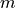previous nodes, chosen with probability: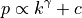where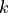is the (in-)degree of the vertex.

We must therefore have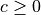for directed graphs and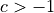for undirected graphs.

If the reciprocity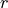is non-zero, each targeted node reciprocates the connection with probability. Expected reciprocity of the final graph is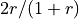.

If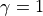, and reciprocity is zero, the tail of resulting in-degree distribution of the directed case is given by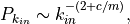or for the undirected case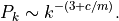However, if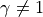, the in-degree distribution is not scale-free.

nngt.generation.random_rewire(g, constraints=None, node_attr_constraints=None, edge_attr_constraints=None, **kwargs)[source]#

Generate a new rewired graph from g.

New in version 2.0.

Parameters:
• g (Graph) – Base graph based on which a new rewired graph will be generated.

• constraints (str, optional (default: no constraints)) – Defines which properties of g will be maintained in the rewired graph. By default, the graph is completely rewired into an Erdos-Renyi model. Available constraints are “in-degree”, “out-degree”, “total-degree”, “all-degrees”, and “clustering”.

• node_attr_constraints (str, optional (default: randomize all attributes)) – Whether attribute randomization is constrained: either “preserve”, where all nodes keep their attributes, or “together”, where attributes are randomized by groups (all attributes of a given node are sent to the same new node). By default, attributes are completely and separately randomized.

• edge_attr_constraints (str, optional (default: randomize all attributes)) – Whether attribute randomization is constrained. If constraints is “in-degree” (respectively “out-degree”) or “degrees”, this can be “preserve_in” (respectively “preserve_out”), in which case all attributes of a given edge are moved together to a new incoming (respectively outgoing) edge of the same node. Regardless of constraints, “together” can be used so that edges attributes are randomized by groups (all attributes of a given edge are sent to the same new edge). By default, attributes are completely and separately randomized.

• **kwargs (optional keyword arguments) – These are optional arguments in the case constraints is “clustering”. In that case, the user can provide both:

• rtol : float, optional (default: 5%) The tolerance on the relative error to the average clustering for the rewired graph.

• connected : bool, optional (default: False) Whether the generated graph should be connected (this reduces the precision of the final clustering).

• method : str, optional (default: “star-component”) Defines how the initially disconnected components of the generated graph should be connected among themselves. Available methods are “sequential”, where the components are connected sequentially, forming a long thread and increasing the graph’s diameter, “star-component”, where all disconnected components are connected to random nodes in the largest component, “central-node” , where all disconnected components are connected to the same node in the largest component, and “random”, where components are connected randomly.

nngt.generation.random_scale_free(in_exp, out_exp, nodes=0, density=None, edges=None, avg_deg=None, reciprocity=0.0, weighted=True, directed=True, multigraph=False, name='RandomSF', shape=None, positions=None, population=None, from_graph=None, **kwargs)[source]#

Generate a free-scale graph of given reciprocity and otherwise devoid of correlations.

Parameters:
• in_exp (float) – Absolute value of the in-degree exponent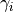, such that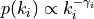• out_exp (float) – Absolute value of the out-degree exponent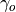, such that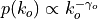• nodes (int, optional (default: 0)) – The number of nodes in the graph.

• density (double, optional) – Structural density given by edges / (nodes*nodes).

• edges (int optional) – The number of edges between the nodes

• avg_deg (double, optional) – Average degree of the neurons given by edges / nodes.

• weighted (bool, optional (default: True)) – Whether the graph edges have weights.

• directed (bool, optional (default: True)) – Whether the graph is directed or not.

• multigraph (bool, optional (default: False)) – Whether the graph can contain multiple edges between two nodes. can contain multiple edges between two

• name (string, optional (default: “ER”)) – Name of the created graph.

• shape (Shape, optional (default: None)) – Shape of the neurons’ environment.

• positions (numpy.ndarray, optional (default: None)) – A 2D or 3D array containing the positions of the neurons in space.

• population (NeuralPop, optional (default: None)) – Population of neurons defining their biological properties (to create a Network)

• from_graph (Graph or subclass, optional (default: None)) – Initial graph whose nodes are to be connected.

Returns:

graph_fs (Graph)

Note

As reciprocity increases, requested values of in_exp and out_exp will be less and less respected as the distribution will converge to a common exponent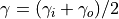. Parameter nodes is required unless from_graph or population is provided.

nngt.generation.sparse_clustered(c, nodes=0, edges=None, avg_deg=None, connected=True, rtol=None, exact_edge_nb=False, weighted=True, directed=True, multigraph=False, name='FC', shape=None, positions=None, population=None, from_graph=None, **kwargs)[source]#

Generate a sparse random graph with given average clustering coefficient and degree.

New in version 2.5.

The original algorithm is adapted from [newman-clustered-2003] and leads to a graph with approximate clustering coefficient and number of edges.

Warning

This algorithm can only give reasonable results for sparse graphs and will raise an error if the requested graph density is above c.

Nodes are distributed among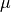overlapping groups of size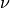and, each time two nodes belong to a common group, they are connected with a probability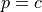.

For sparse graphs, the average (in/out-)degree can be approximmated as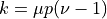, and the average clustering as: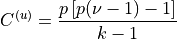for undirected graphs and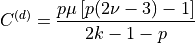for all clustering modes in directed graphs.

From these relations, we computeandas: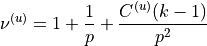or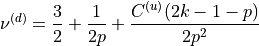and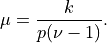Parameters:
• c (float) – Desired value for the final average clustering in the graph.

• nodes (int, optional (default: None)) – The number of nodes in the graph.

• edges (int, optional) – The number of edges between the nodes

• avg_deg (double, optional) – Average degree of the neurons given by edges / nodes.

• connected (bool, optional (default: True)) – Whether the resulting graph must be connected (True) or may be unconnected (False).

• rtol (float, optional (default: not checked)) – Tolerance on the relative error between the target clustering c and the actual clustering of the final graph. If the algorithm leads to a relative error greater than rtol, then an error is raised.

• exact_edge_nb (bool, optional (default: False)) – Whether the final graph should have precisely the number of edges required.

• weighted (bool, optional (default: True)) – Whether the graph edges have weights.

• directed (bool, optional (default: True)) – Whether the graph should be directed or not.

• multigraph (bool, optional (default: False)) – Whether the graph can contain multiple edges between two nodes.

• name (string, optional (default: “ER”)) – Name of the created graph.

• shape (Shape, optional (default: None)) – Shape of the neurons’ environment.

• positions (numpy.ndarray, optional (default: None)) – A 2D or 3D array containing the positions of the neurons in space.

• population (NeuralPop, optional (default: None)) – Population of neurons defining their biological properties (to create a Network).

• from_graph (Graph or subclass, optional (default: None)) – Initial graph whose nodes are to be connected.

• **kwargs (keyword arguments) – If connected is True, method can be passed to define how the components should be connected among themselves. Available methods are “sequential”, where the components are connected sequentially, forming a long thread and increasing the graph’s diameter, “star-component”, where all components are connected to the largest one, and “random”, where components are connected randomly, or “central-node”, where one node from the largest component is chosen to reconnect all disconnected components. If not provided, defaults to “random”.

Note

nodes is required unless from_graph or population is provided. If from_graph is provided, all preexistent edges in the object will be deleted before the new connectivity is implemented.

Returns:

graph_fc (Graph, or subclass) – A new generated graph or the modified from_graph.

References

Newman, M. E. J. Properties of Highly Clustered Networks, Phys. Rev. E 2003 68 (2). DOI: 10.1103/PhysRevE.68.026121, arXiv: cond-mat/0303183.

nngt.generation.watts_strogatz(coord_nb, proba_shortcut=None, reciprocity_circular=1.0, reciprocity_choice_circular='random', shuffle='random', nodes=0, weighted=True, directed=True, multigraph=False, name='WS', shape=None, positions=None, population=None, from_graph=None, **kwargs)[source]#

Generate a (potentially small-world) graph using the Watts-Strogatz algorithm.

For directed networks, the reciprocity of the initial circular network can be chosen.

New in version 2.0.

Parameters:
• coord_nb (int) – The number of neighbours for each node on the initial topological lattice (must be even).

• proba_shortcut (double, optional) – Probability of adding a new random (shortcut) edge for each existing edge on the initial lattice. If edges is provided, then will be computed automatically as edges / (coord_nb * nodes * (1 + reciprocity_circular) / 2)

• reciprocity_circular (double, optional (default: 1.)) – Proportion of reciprocal edges in the initial circular graph.

• reciprocity_choice_circular (str, optional (default: “random”)) – How reciprocal edges should be chosen in the initial circular graph. This can be either “random” or “closest”. If the latter option is used, then connections between first neighbours are rendered reciprocal first, then between second neighbours, etc.

• shuffle (str, optional (default: ‘random’)) – Whether to shuffle only ‘targets’ (out-degree of all nodes remains constant), ‘sources’ (in-degree remains constant), or randomly the source or the target for each edge (‘random’) in the case of directed graphs.

• nodes (int, optional (default: None)) – The number of nodes in the graph.

• weighted (bool, optional (default: True)) – Whether the graph edges have weights.

• directed (bool, optional (default: True)) – Whether the graph is directed or not.

• multigraph (bool, optional (default: False)) – Whether the graph can contain multiple edges between two nodes.

• name (string, optional (default: “ER”)) – Name of the created graph.

• shape (Shape, optional (default: None)) – Shape of the neurons’ environment

• positions (numpy.ndarray, optional (default: None)) – A 2D or 3D array containing the positions of the neurons in space.

• population (NeuralPop, optional (default: None)) – Population of neurons defining their biological properties (to create a Network).

• from_graph (Graph or subclass, optional (default: None)) – Initial graph whose nodes are to be connected.

Returns:

graph_nw (Graph` or subclass)

Note

nodes is required unless from_graph or population is provided.